Equation graph plotter - EqPlot 1.2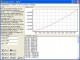Equation graph plotter software plots 2D graphs from complex mathematical equations.

 Developer: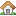Institute of Mathematics and Statistics      software by Institute of Mathematics and Statistics → Price: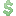15.00  buy → License: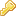Shareware File size: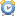0K Language: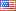OS: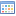Windows Vista (?) Rating: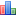0 /5 (0 votes)

Equation graph plotter software plots 2D graphs from complex mathematical equations. The application comprises algebraic, trigonometric, hyperbolic and transcendental functions.

EqPlot can be used to verify the results of nonlinear regression analysis program.

Graphically Review Equations:
Equation graph plotter gives engineers and researchers the power to graphically review equations, by putting a large number of equations at their fingertips. The program is also indispensable for students and teachers.

Understandable and convenient interface:
A flexible work area lets you type in your equations directly. It is as simple as a regular text editor. Annotate, edit and repeat your graphings in the work area. You can also paste your equations into the editor panel.
Example of mathematical expression:
5.22 - (2 * x) + square(x) + power(x;3) + power(2.55;4) - logbaseN(4;6.25)
Save your work for later use into a text or graphic file. Comprehensive online help is easily accessed within the program.

Here are some key features of "Equation graph plotter EqPlot":

• Scientific graphings
• Unlimited expression length
• Parenthesis compatible
• Scientific notation
• More than 35 functions
• More than 40 constants
• User-friendly error messages
• Simple mode (medium size on desktop)
• Paste express

tags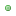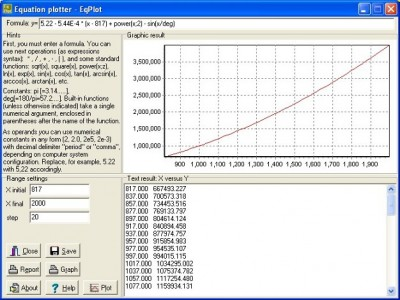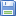Download Equation graph plotter - EqPlot 1.2

Purchase: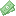Buy Equation graph plotter - EqPlot 1.2

Authors softwareEquation graph plotter - EqPlot 1.2
Institute of Mathematics and Statistics

Equation graph plotter software plots 2D graphs from complex mathematical equations.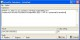Scientific calculator - ScienCalc 1.3.7
Institute of Mathematics and Statistics

Scientific calculator - ScienCalc software represents a convenient and powerful scientific calculator.Nonlinear regression analysis - CurveFitter 1.1
Institute of Mathematics and Statistics

Nonlinear regression analysis - CurveFitter software performs statistical regression analysis to estimate the values of parameters for linear, multivariate, polynomial, exponential and nonlinear functions.

Similar softwareEquation graph plotter - EqPlot 1.2
Institute of Mathematics and Statistics

Equation graph plotter software plots 2D graphs from complex mathematical equations.Scientific calculator - ScienCalc 1.3.7
Institute of Mathematics and Statistics

Scientific calculator - ScienCalc software represents a convenient and powerful scientific calculator.Graph FAWM 1.3
New FAWM Creations

Graph FAWM application is an equation plotter designed for use by students and teachers of mathematics.Nonlinear regression analysis - CurveFitter 1.1
Institute of Mathematics and Statistics

Nonlinear regression analysis - CurveFitter software performs statistical regression analysis to estimate the values of parameters for linear, multivariate, polynomial, exponential and nonlinear functions.Ordinary Differential Equations 1.00
Orlando Mansur

Ordinary Differential Equations solves boundary-value or initial-value problems involving nonlinear or linear ordinary differential equations of any order, or systems of such.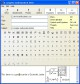Scientific Letter 1.90.00
Scientific Networks Software

Scientific Letter is an original mail program that allows you to create messages with complex equations.Systems of Nonlinear Equations 1.00
Orlando Mansur

System of Nonlinear Equations application numerically solves systems of simultaneous nonlinear equations.A&G Grapher 5.6
Runiter

Graphing equations has never been any easier! A&G Grapher program is as easy to use as typing down the desired equation (e.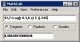MathCalc 2.1
YPS software

MathCalc is a powerful mathematical calculator as well as easy-to-use 2D graph plotter.Graph Plotter 1.2.3
Still Soft

Graph Plotter is a powerful utility that will help you plot and analyze different types of graphs.

Other software in this categoryEye Relax 1.2
United Research Labs

Everyday so many thousands of people across the globe are working on their computer for hours.DANCE - the dance patterns database 4.51

DANCE - the dance patterns database is a very useful utility for those who want to learn how to dance.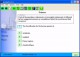Academia 3.0
Genesis Software

Academia is an educational program that can be used by anyone.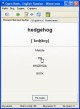Open Book 2.3.4
Aleksei Vinidiktov

Open Book is a useful vocabulary maker utility for Windows 98, Me, XP, 2000, 2003 Server featuring an effective method for memorizing information.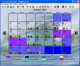Hormonal Forecaster - Fertility Software 5.2
Brian Frackelton

The Hormonal Forecaster easily charts fertility and ovulation to help you avoid or achieve pregnancy and conception by charting the most fertile days of a woman`s menstruation.